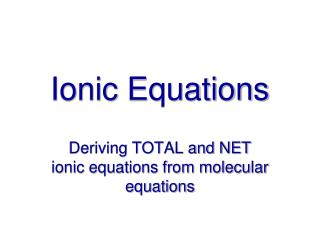DownloadDownload PresentationIonic Equations

# Ionic Equations

Télécharger la présentation## Ionic Equations

- - - - - - - - - - - - - - - - - - - - - - - - - - - E N D - - - - - - - - - - - - - - - - - - - - - - - - - - -
##### Presentation Transcript

1. Ionic Equations Deriving TOTAL and NET ionic equations from molecular equations

2. Molecular equations • Show all substances as elements or compounds using appropriate formulae • Identify as solid (s), gas (g), liquid (l) or dissolved (aq) • In these equations essential to show states Pb(NO3)2(aq) + 2KI(aq) = 2KNO3(aq) + PbI2(s)

3. Total ionic equations • For all dissolved substances show as constituent ions (except weak acids or other weak electrolytes) • All others show as molecular formula Pb(NO3)2(aq) + 2KI(aq) = 2KNO3(aq) + PbI2(s) Pb2+(aq) + 2NO3-(aq) + 2K+(aq) + 2I-(aq) = 2K+(aq) + 2NO3-(aq) + PbI2(s)

4. Net ionic equations • Spectator ions are those ions that do not undergo a change; they do not participate in the chemical change and are the same on both sides of the equation • Remove all spectator ions from the equation Pb2+(aq) + 2NO3-(aq) + 2K+(aq) + 2I-(aq) = 2K+(aq) + 2NO3-(aq) + PbI2(s)

5. Net ionic equations Pb2+(aq) + 2I-(aq) = PbI2(s) • Mass and charge must still balance, although overall charge may not be neutral in a net ionic equation

6. Strong electrolytes are ionized, weak electrolytes are not